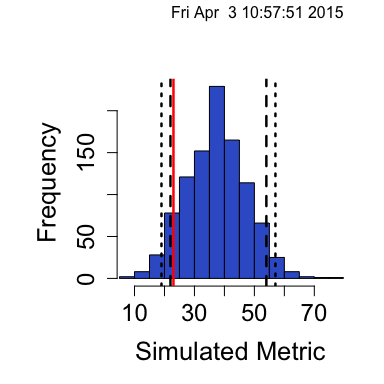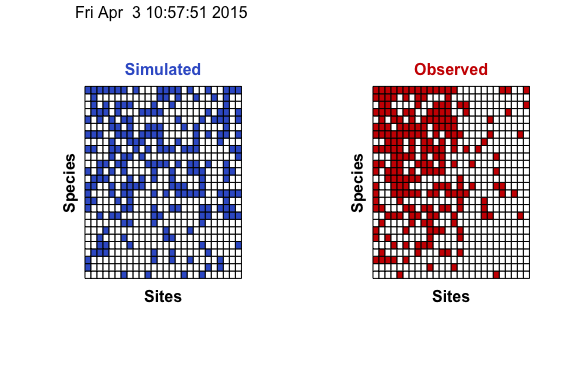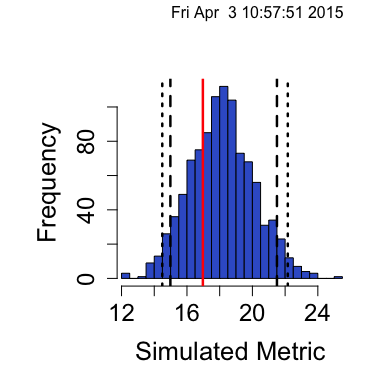## Introduction

EcoSimR provides a variety of options to fit niche-overlap, size ratio and co-occurrence null models with algorithms and metrics out of the box. Each of these are easily callable with niche_null_model(),size_null_model(), or cooc_null_model(). These functions are actually just wrappers for a function called null_model_engine(). The wrappers are conveniences that control inputs, and provide easy summary and plotting functions. However you can add your own algorithm and metrics. These can be completely novel or can be used with an existing null model. The first part is defining a metric or algorithm function that is compatible with the null model engine. This is because each user defined function requires a standard set of inputs to work with the null model engine.

## User Defined Function Overview

Algorithms

Algorithms in the context of the null model engine are any function that will randomize an input data frame and return that randomization in the same form of the input. The classic example is of a co-occurrence null model. The input is a data frame of 1’s and 0’s that reperesent a species x site co-occurrence matrix where a 1 or 0 represents incidence of Species 1 at Site 1 (and so forth). A suitable randomization algorithm will accept this input and return a randomized matrix of the same size. All user defined algorithm require that the first parameter be called speciesData and is the species data to randomize e.g.:

foo <- function(speciesData,...){
## Code to randomize here!
}

A simple example might look like this (this is actually sim1() )

myAlgo <- function(speciesData) {
return(matrix(sample(speciesData), ncol=ncol(speciesData)))
}

Following the required speciesData parameter, you can include an arbitrary number of parameters. Here’s an example (actually sim10() )

myAlgo2 <- function(speciesData,rowWeights,colWeights) {
matrixWeights <- outer(rowWeights,colWeights)
return(matrix(vector_sample(speciesData, weights =matrixWeights),ncol=ncol(speciesData)))
} 

Metrics

A user defined metric function will take data frame and calculate a scalar value. The null model distribution is the distribution of these values. Similar to algorithms, metrics require that the first parameter be called m. Here’s a simple example (species_combo() in the package).

bar <- function(m){
return(ncol(unique(m,MARGIN=2)))
}

Also an arbirtary number of parameters can be added with any names you choose.

bar <- function(m, trim = FALSE){
if(trim){
m <- m[which(rowSums(m)>0),]
}
return(ncol(unique(m,MARGIN=2)))
}

Now that user defined functions have been written they can easily be plugged into the null model engine.

## Adding to existing null models

One use case for creating your own algorithms and metrics is because you want to plug into the existing null model frame works in the package. Let’s use co-occurrence models as an example. The cooc_null_model() function provides an easy interface with existing models, but power users may want to build upon the provided metrics and algorithms. First create a user defined algorithm.

myAlgo <- function(speciesData) {
matrixWeights <- outer(rowSums(speciesData),rep(1,ncol(speciesData)))
return(matrix(vector_sample(speciesData, weights=matrixWeights),ncol=ncol(speciesData)))
}

Now choose an existing metric you want to use, for this example let’s choose the checker metric. Because we want to have our results be compatible with an existing co-occurrence model, we need to specify the type of model we’re running. The options are

• cooc for co-occurrence models
• niche for niche overlap models
• size for size ratio models
## Simulate data
coocSimData <- ranMatGen(aBetaCol=0.5,bBetaCol=0.5, aBetaRow=0.5,bBetaRow=0.5, numRows=30,numCols=30,
mFill=0.25,abun=0,emptyRow=FALSE, emptyCol=FALSE)\$m

coocOut <- null_model_engine(speciesData = coocSimData,nReps=1000,
algo = "myAlgo", metric = "checker",type="cooc")

This will create a co-occurrence null model with your own alogrithm, and use the checker metric. The advantage of this is that you can now make co-occurrence plots and compare to existing null models.

## Histogram plots
plot(coocOut,type='hist')## Matrix examples
plot(coocOut, type='cooc')## Summary function
summary(coocOut)
## Time Stamp:  Fri Apr  3 10:57:51 2015
## Reproducible:  FALSE
## Number of Replications:  1000
## Elapsed Time:  2.9 secs
## Metric:  checker
## Algorithm:  myAlgo
## Observed Index:  23
## Mean Of Simulated Index:  37.867
## Variance Of Simulated Index:  100.63
## Lower 95% (1-tail):  22
## Upper 95% (1-tail):  54
## Lower 95% (2-tail):  19
## Upper 95% (2-tail):  57.025
## Lower-tail P =  0.08
## Upper-tail P =  0.938
## Observed metric > 62 simulated metrics
## Observed metric < 920 simulated metrics
## Observed metric = 18 simulated metrics
## Standardized Effect Size (SES):  -1.482

## Creating novel null models

You can create novel null models in a similar manner. Instead of just adding your own new algorithm and using an existing metric, you just create a new algorithm and a metric. If you do this, there’s no need to specify the type. However if your new alogrithm and metric combination work on the same data as a co-occurrence model, you could specify the type as cooc. Specifying the type simply allows you to make type specific plots.

First we’ll create new algorithms and new metrics. We’ll also create an algorithm that has extra parameters so you can see how easy it is to add custom parameters.

myAlgo <- function(speciesData,rowWeights,colWeights) {
matrixWeights <- outer(rowWeights,colWeights)
return(matrix(vector_sample(speciesData, weights =matrixWeights),ncol=ncol(speciesData)))
}

## The C-Score
myMetric <- function(m)
{
m <- m[which(rowSums(m)>0),] # make calculation on submatrix with no missing species
shared = tcrossprod(m)
sums = rowSums(m)

upper = upper.tri(shared)

scores = (sums[row(shared)[upper]] - shared[upper])*
(sums[col(shared)[upper]] - shared[upper])

return(mean(scores))
}

It’s easy to plug our new metric and algorithm into the null model engine. Note that at the end we have a list of parameter options. This is how you can pass any extra parameters to your algorithms or metrics.

novelNull <- null_model_engine(coocSimData,algo="myAlgo",metric="myMetric",
algoOpts = list(rowWeights = runif(dim(coocSimData)),
colWeights = runif(dim(coocSimData))))

Your new null model can produce a similar summary to the standard null models, as well as a basic histogram plot.

summary(novelNull)
## Time Stamp:  Fri Apr  3 10:57:51 2015
## Reproducible:  FALSE
## Number of Replications:  1000
## Elapsed Time:  0.4 secs
## Metric:  myMetric
## Algorithm:  myAlgo
## Observed Index:  16.972
## Mean Of Simulated Index:  18.147
## Variance Of Simulated Index:  3.839
## Lower 95% (1-tail):  14.986
## Upper 95% (1-tail):  21.501
## Lower 95% (2-tail):  14.491
## Upper 95% (2-tail):  22.163
## Lower-tail P =  0.275
## Upper-tail P =  0.725
## Observed metric > 275 simulated metrics
## Observed metric < 725 simulated metrics
## Observed metric = 0 simulated metrics
## Standardized Effect Size (SES):  -0.59942
plot(novelNull)Any randomization algorithm and metric can be plugged into the null model framework. You can also set the type of null model you create to easily print summaries and plots to compare to the existing null models available in EcoSimR.